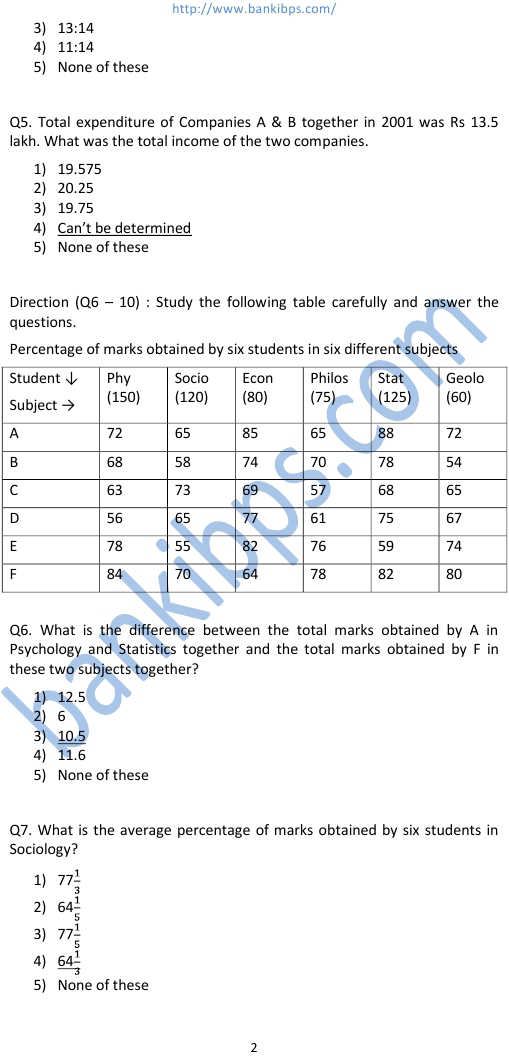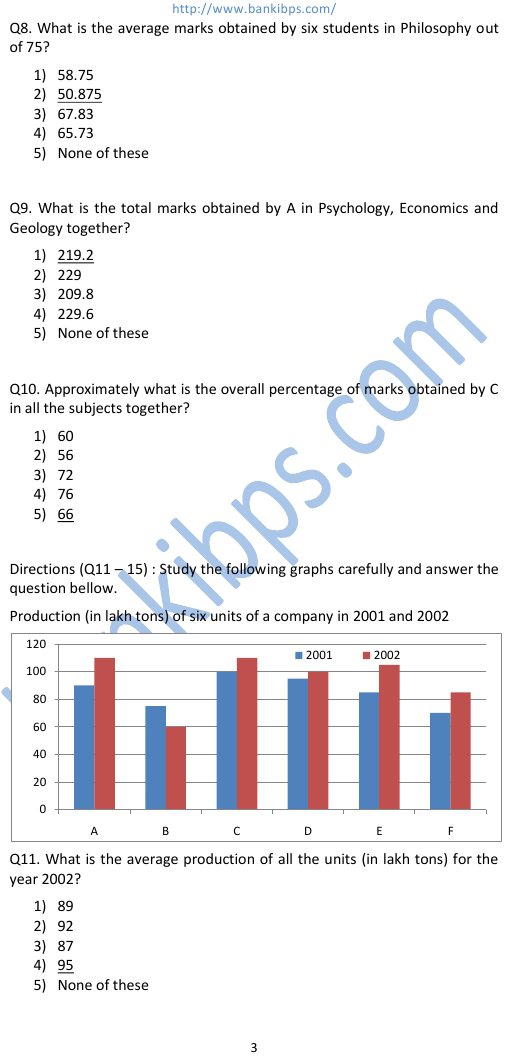# Data Analysis and Sufficiency

Q1. If the income of Company A in 1998 was Rs. 142500 what was its expenditure in that year? data analysis and sufficiency 1) Rs 105000 2) Rs 95500 3) Rs 99500 4) Rs 105555 5) None of these Q2. Expenditure of Company B in 1999 was 90% of its expenditure in 1999. Income of Company B in 1999 was what percent of its income in 1998? 1) 130.5 2) 96.66 3) 121.5 4) 99.33 5) None of these Q3. If the expenditure of company A in 1997 was Rs 70 lakhs and income of Company A in 1997 was equal to its expenditure in 1998, what was the total income (in Rs lakh ) of the Company A in 1997 & 1998 together? 1) 175 2) 131.25 3) 218.75 4) Can’t be determined 5) None of these
Practice Exercise - 229 [ Data Analysis ]## data analysis and sufficiency

### data analysis and sufficiency questions

#### data analysis and sufficiency for mat

##### data sufficiency question
###### data analysis powerpoint
data analysis and sufficiency questions. data analysis and sufficiency for mat. data sufficiency questions. data sufficiency question. data analysis test. data analysis questions for mba. data analysis powerpoint. data analysis practice. data analysis tests. free online data analysis. mba data analysis. data interpretation and data sufficiency.